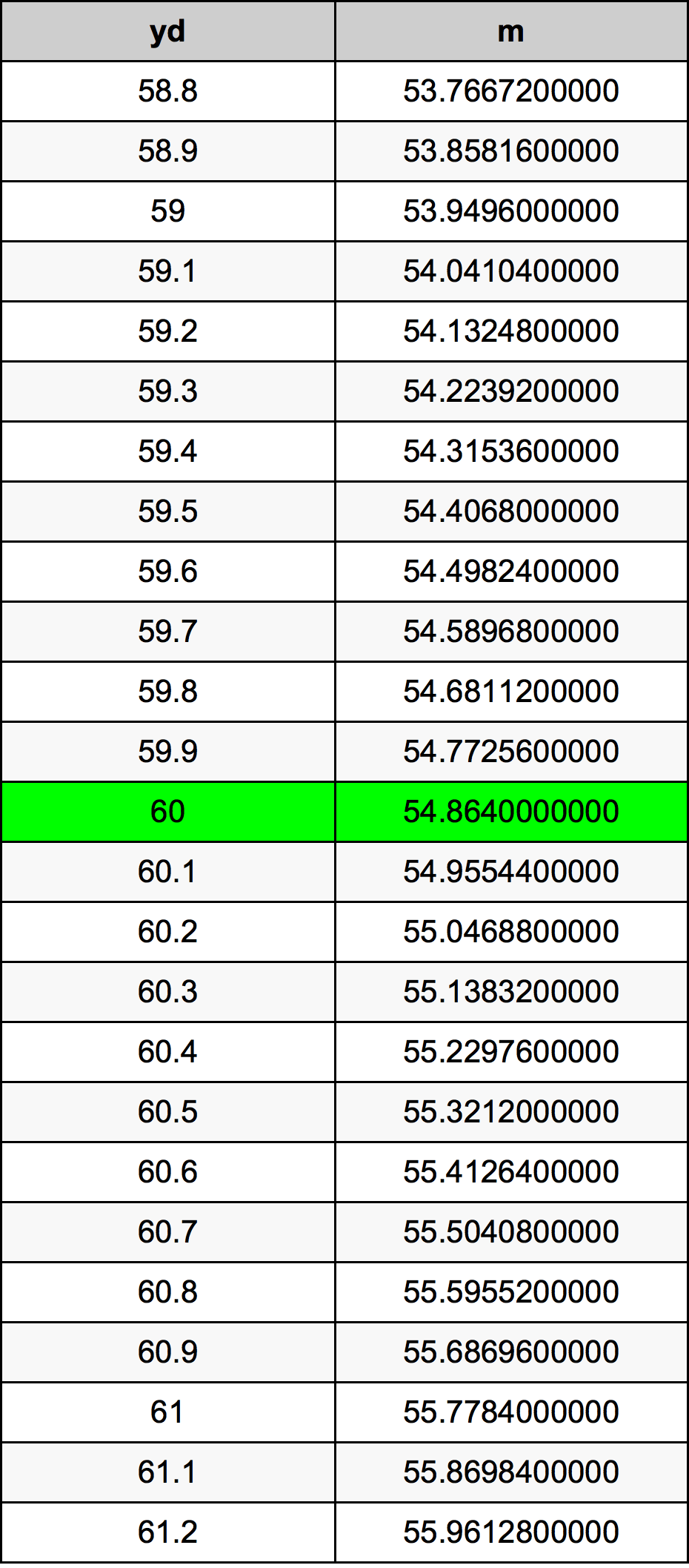Yards To Meters

# 60 yd to m60 Yards to Meters

yd
=
m

## How to convert 60 yards to meters?

 60 yd * 0.9144 m = 54.864 m 1 yd
A common question is How many yard in 60 meter? And the answer is 65.6167979003 yd in 60 m. Likewise the question how many meter in 60 yard has the answer of 54.864 m in 60 yd.

## How much are 60 yards in meters?

60 yards equal 54.864 meters (60yd = 54.864m). Converting 60 yd to m is easy. Simply use our calculator above, or apply the formula to change the length 60 yd to m.

## Convert 60 yd to common lengths

UnitLengths
Nanometer54864000000.0 nm
Micrometer54864000.0 µm
Millimeter54864.0 mm
Centimeter5486.4 cm
Inch2160.0 in
Foot180.0 ft
Yard60.0 yd
Meter54.864 m
Kilometer0.054864 km
Mile0.0340909091 mi
Nautical mile0.0296241901 nmi

## What is 60 yards in m?

To convert 60 yd to m multiply the length in yards by 0.9144. The 60 yd in m formula is [m] = 60 * 0.9144. Thus, for 60 yards in meter we get 54.864 m.

## 60 Yard Conversion Table## Alternative spelling

60 Yard to Meter, 60 Yard in Meter, 60 yd to m, 60 yd in m, 60 Yard to m, 60 Yard in m, 60 Yard to Meters, 60 Yard in Meters, 60 Yards to m, 60 Yards in m, 60 Yards to Meters, 60 Yards in Meters, 60 yd to Meter, 60 yd in Meter